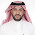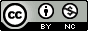## Friday, October 9, 2009

### Visualizing sample relatedness in a GWAS using PLINK and R

Strict quality control procedures are extremely important for any genome-wide association study.  One of the first steps you should take when running QC on your GWAS is to look for related samples in your dataset.  This does two things for you.  First, you can get an idea of how many related samples you have in your dataset, and second, if you have access to self-report relationship information, you can identify potential sample mix-ups based on discrepancies between genetic information and self-report.

PLINK allows you to estimate genomewide IBD-sharing coefficients between seemingly unrelated individuals from whole-genome data.  You can read lots more about the particular method they use to infer IBD given IBS and allele frequencies in the PLINK paper.  It's relatively straightforward to compute these estimates.  If you already have your data in pedfile format, use the following command (assuming mydata.ped and mydata.map are in the current directory where you run PLINK).

plink --file mydata --genome --min 0.05

This will usually take a few days if you use genome-wide data.  You could speed this up by first thinning your dataset to around 100,000 markers using the --thin option to create a smaller dataset.

After you've run this you'll have a file in that directory called plink.genome.  You can read about the output here, but we're really interested in Z1 and Z0, the proportion of markers identical by descent 1 and 0 respectively, for every pair of individuals in the dataset.  You can plot Z1 by Z0 and color code them by the relationship type as coded in the pedfile using this R code.

Here's what the columns of interest will look like:

```  RT     Z1     Z0
1 UN 0.1697 0.8294
2 OT 0.5034 0.4879
3 OT 0.4403 0.5439
4 OT 0.5200 0.4674
5 UN 0.1646 0.8311
6 OT 0.5519 0.4481

```

First, fire up R, go to the directory where your plink results are located and load in the data:

Next, set up the plot area. The par option makes the points solid instead of an open circle, and the plot command sets up your plot and axes without adding any points.

par(pch=16)
with(d,plot(Z0,Z1, xlim=c(0,1), ylim=c(0,1), type="n"))

Your plot should be empty, like this:

Now use this command to plot points where the relationship type is FS, or full sibling.  Make the points green (col=3).

with(subset(d,RT=="FS") , points(Z0,Z1,col=3))

Do the same thing with half sibs, "other related" (have the same family ID), parent offspring pairs, and unrelated, making each of them a different color.

with(subset(d,RT=="HS") , points(Z0,Z1,col="darkorange"))
with(subset(d,RT=="OT") , points(Z0,Z1,col=4))
with(subset(d,RT=="PO") , points(Z0,Z1,col=2))
with(subset(d,RT=="UN") , points(Z0,Z1,col=1))

You might want to rerun the commands for "other related" and especially half sibs, because they were covered up when you plotted unrelateds.

with(subset(d,RT=="OT") , points(Z0,Z1,col=4))
with(subset(d,RT=="HS") , points(Z0,Z1,col="darkorange"))

legend(1,1, xjust=1, yjust=1, legend=levels(d\$RT), pch=16, col=c(3,"darkorange",4,2,1))

For a recap, your code should look like this:

par(pch=16)
with(d,plot(Z0,Z1, xlim=c(0,1), ylim=c(0,1), type="n"))

with(subset(d,RT=="FS") , points(Z0,Z1,col=3))
with(subset(d,RT=="HS") , points(Z0,Z1,col="darkorange"))
with(subset(d,RT=="OT") , points(Z0,Z1,col=4))
with(subset(d,RT=="PO") , points(Z0,Z1,col=2))
with(subset(d,RT=="UN") , points(Z0,Z1,col=1))
with(subset(d,RT=="OT") , points(Z0,Z1,col=4))
with(subset(d,RT=="HS") , points(Z0,Z1,col="darkorange"))

legend(1,1, xjust=1, yjust=1, legend=levels(d\$RT), pch=16, col=c(3,"darkorange",4,2,1))

What you can see from the above plot is that self-reported parent-offspring pairs share 100% of their alleles IBD=1.  There is a single sib pair at the bottom left where Pr(IBD=1)=0 and Pr(IBD=0)=0.  This means that this sib pair shares 2 alleles identical by descent at every locus across the genome.  This is either a duplicated sample or a set of identical twins.  The full sibs cluster right around the middle of the plot, and you have lots of unrelated or "other related" pairs stretching from completely unrelated (bottom right quadrant) to relatively closely related (2nd or 3rd degree relatives, right around the middle of the plot).  If you see self-reported sibs showing up where the unrelated individuals cluster on this plot, or vice versa, this should clue you in on potential sample identity problems.

...

Just for fun, let's make the same plot using ggplot2.  Make sure you have the data loaded as above, then install ggplot2 if you haven't already

install.packages("ggplot2")

You only have to install the package once.  Next load the package:

library(ggplot2)

Use this incredibly simple command to make the plot:

qplot(Z0,Z1,data=d,colour=RT)

I'm not a huge fan of these colors, so lets make them the same as above:

qplot(Z0,Z1,data=d,colour=RT) + scale_colour_manual(value=c(3,"darkorange",4,2,1))

That's a little better.  Obviously the code to do this is MUCH simpler in ggplot2.  The only problem I have with this is that it automatically plots the points in the order of the levels of RT, ie. FS, then HS, then OT, PO, then UN, so you end up covering up all your half-sibs with "other related" and unrelated pairs.  Post a comment if you know how to go back and replot the HS points over the UN points.  For more on ggplot2, see my previous post comparing histograms made using Stata, R base, R lattice and R ggplot2.

1.It was great to follow your demnstration about ggplot2 using IBD/IBS (plink out put file of pair-wise IBD distance),then showed how plot ggplot2 using R - great staff!

I am interesting in to reproduce similar plot. Can you please direct me where I can get a data to practice?

Cheers,
Ali
abdirahman.ali@sydney.edu.au

2.May I ask what cut-off of PI-HAT should be used to distinguish the completely unrelated from the relatively related subjects based on the genotyping data?

Thanks very much!
Dan
simpledandan@gmail.com

3.Hmm where did the images go?

1.Unfortunately I had these images hosted at Vanderbilt, and when I left, they disappeared. For the time being, you can find the images cross-posted at the eMERGE network, but I will try to fix the images here soon.

4.Looks like 'value' should now be 'values' in this:

> qplot(Z0,Z1,colour=RT) + scale_colour_manual(value=c(3,"darkorange",4,2,1))
Error in structure(list(call = match.call(), aesthetics = aesthetics, :
argument "values" is missing, with no default
> qplot(Z0,Z1,colour=RT) + scale_colour_manual(values=c(3,"darkorange",4,2,1))

5.Yes, ggplot2 has undergone some changes since I wrote this. I need to rerun this code and fix the broken links.

6.it's possible call plink package inside R?

1.7.Reading the .genome file from R taking so much time.. is there any other way to reduce the time or I should wait?

1.When you calculate IBD, you can filter out low values from the result by setting --min 0.05 (for example). This prevents you from having N^2 results, and only finding pairwise results that have some meaningful level of IBD.